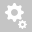##Recently viewed tickets# Combined stress for moving load

Question:

Hi,

I want to get clarification on the combined moving load stress to composite section.

As show in attached mode, I checked the max tension stress at element 1314 under moving load case "Cen MV". The combined stress at point 4(-y,-z) is 11.1MPa, however, the individual stresses are 2.99MP for Sax, 0.795MPa for Sby, 5.72MPa for Sbz. All this stress will give sum as 9.505MPa. It's different to the combined stress.

I also extract the critical moving load location as "MVmaxCenMVMy1314" static loadcase. under this loadcase, the combined stress at 4(-y,-z) is 8.3MPa, whick is closer to 9.505MPa.

Could you please advise where I am wrong?Answer:

Actually, this issue happened because of the unsymmetrical section. Let me explain this by the following example:-In the bending stress column, the program would show the maximum possible stress component of the section. For Mz in the above section maximum stress would occur at points 1 & 2. Therefore in bending stress column, it shows this stress result.Now for the combined stress calculation at points 3 & 4, we can not simply add this stress to the axial stress. The program internally calculates the stress at point 3 & 4 for the Mz component and then calculate the combined stress. I have attached a verification excel sheet for your reference.

Hope this helps. Kindly let us know if further assistance would be required.

Regards,
Kaushal Mittal

Hi Kaushal,

Thanks for your response.

I agree with you when the combined stress is calculated for a static load case. As stated in my question, static load case " MVmaxCenMVMy1314" follows this well: 2.9+0.127+5.4=8.427MPa, which corresponds to Cb4 point stress well (8.34MPa).

However, for the moving load case "Cen MV(max)", the combined stress gives 2.99+0.795+5.72=9.509MPa, which is much lower than Cb4 point stress (11.2MPa).

The static load case "MVmaxCenMVMy1314" is the critical load case for element 1314 which I used moving load tracer to account for the max stress at element 1314. It can be seen the Sax, My-, and Mz- stress are pretty close between "MVmaxCenMVMy1314" and "Cen MV(max)", but the combined stress differs a lot.

From you explanation, I believe the "MVmaxCenMVMy1314" load case gives right combined stress, but the moving load case might not be right.

Could you please give it a further look?

Regards

This is happening because for the moving load case the program gives us the envelope results. Let me explain this by the following example:-

In combined stress, there are 3 components, A(axial stress), B (Bending -Y stress), and C (Bending - Z stress. Now to maximize the A+B+C, I have taken three static load case (created using MVL tracer):- (1) Amax & concurrent (2) Bmax & concurrent (3) Cmax & concurrent.But still, the stress results we got from the MIDAS are more from my calculated results. This happened because (A+B+C) max can occur at any other case in which all 3 (A, B, C) are not at its maximum individually but the submission is at the maximum. The program internally considers all the cases as per the influence line and gives us the result of the critical case. Unfortunately, the moving load tracer option is not available for the stresses (composite section) therefore exact static load cases can not be created for this.

Hope this helps. Kindly let us know if further assistance would be required.

Regards,
Kaushal Mittal

Kindly find the excel sheet and sample model for reference.

Regards,
Kaushal Mittal
Creation date: 11/27/2020 6:18 AM (Kaushal Mittal)      Updated: 11/30/2020 11:28 AM (dklee@midasit.com)